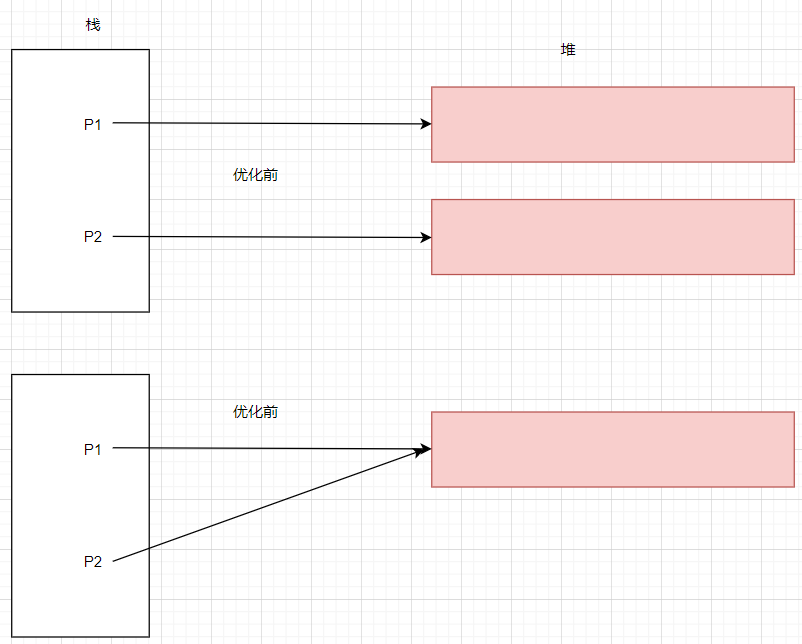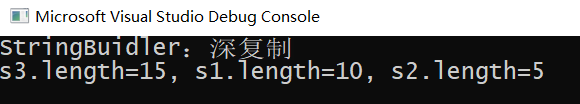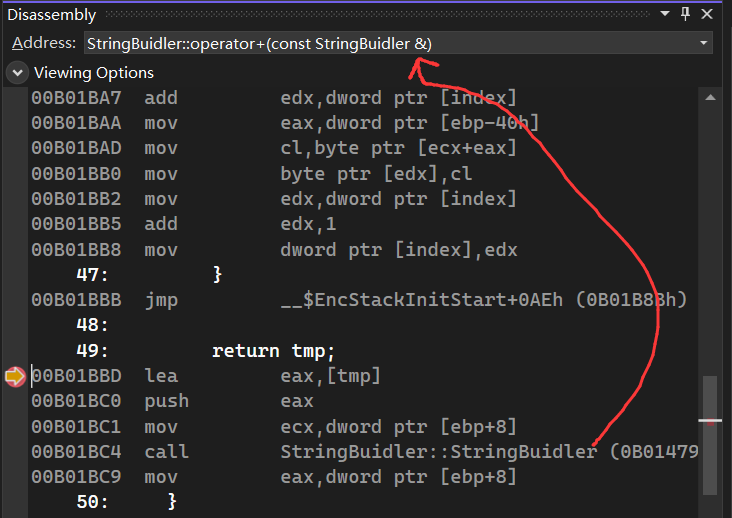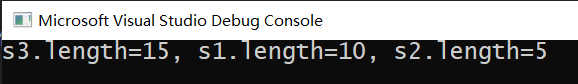## 二： 右值引用

### 2. 右值引用是个什么样子？

``````
int main()
{
int i = 10;
int j = 11;

int sum = i + j;
}

``````
1. 10，11，(i+j)

1. i,j,sum

``````
int main()
{
int i = 10;
int& k = i;		//左值引用

int&& m = 10;	//右值引用
}

``````

``````
33: 	int i = 10;
00FB182F  mov         dword ptr [ebp-0Ch],0Ah
34: 	int& k = i;
00FB182F  mov         dword ptr [ebp-0Ch],0Ah
00FB1836  lea         eax,[ebp-0Ch]
00FB1839  mov         dword ptr [ebp-18h],eax
36: 	int&& m = 10;
00FB183C  mov         dword ptr [ebp-30h],0Ah
00FB1843  lea         eax,[ebp-30h]
00FB1846  mov         dword ptr [ebp-24h],eax

``````

## 三： 右值引用如何减少对象的创建

### 1. 简要思路### 2. 一个简单的例子

C++ 最烦的地方就是有太多的`构造函数`, 数不胜数，太尴尬了，这里我做一个简单的 `+` 操作例子。

``````

#include <iostream>
#include <vector>

using namespace std;

class StringBuidler {
public:
char* str;
int length;
public:
StringBuidler() {}
StringBuidler(int len, char c) {
this->str = new char[len];
this->str = c;
this->length = len;
}

StringBuidler(const StringBuidler& s) {

printf("StringBuidler：深复制 \n");
this->length = s.length;
this->str = new char[s.length];

for (size_t i = 0; i < length; i++)
{
this->str[i] = s.str[i];
}
}

StringBuidler operator+(const StringBuidler& p) {

StringBuidler tmp;

tmp.length = this->length + p.length;
tmp.str = new char[tmp.length];

int index = 0;

for (size_t i = 0; i < this->length; i++)
{
tmp.str[index++] = this->str[i];
}
for (size_t i = 0; i < p.length; i++)
{
tmp.str[index++] = p.str[i];
}

return tmp;
}
};

int main()
{
StringBuidler s1(10, 'a');
StringBuidler s2(5, 'b');

StringBuidler s3 = s1 + s2;

printf("s3.length=%d, s1.length=%d, s2.length=%d \n", s3.length, s1.length, s2.length);
}

``````### 3. 性能优化方案

``````
StringBuidler(StringBuidler&& s) {
this->str = s.str;
this->length = s.length;

s.str = nullptr;
}

``````